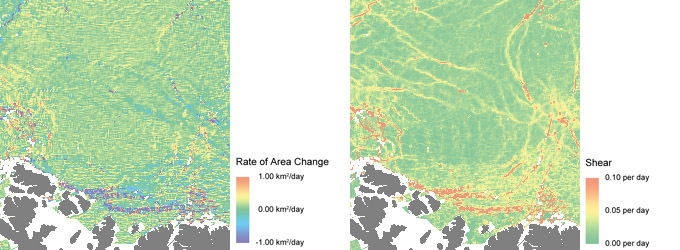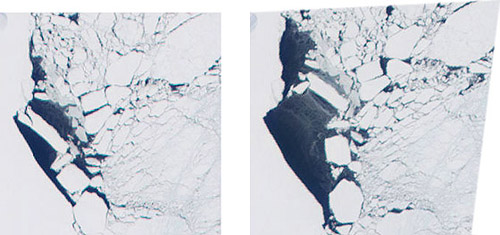Data Products
Ice Deformation

GlobICE product names: DTO3DAY5KM, DTOMON20KM, DTOMON100K

An initially regular grid with vertices tracking sea ice features becomes deformed as the ice moves from day to day. (animation courtesy Ron Kwok, JPL)

By considering the displacement vectors for four adjacent grid points defining a rectangle (or grid cell), measures of ice deformation are calculated and recorded in the Combined Ice Deformation (Thickness) and Open Water Fraction (DTO) Product, available for 3 day intervals on a 5km grid, and monthly intervals on 20km and 100km grids.

Deformation Components

Ice Deformation is characterised by rate of area change, shear rate, vorticity rate and divergence rate. Area change is simply the difference between the area of a cell at the start and end of the time interval. Shear is the change of angle between two initially perpendicular cell boundary lines. Vorticity of a cell is the average angular velocity of two initially parallel cell boundary lines. Divergence is the rate at which two initially parallel cell boundary lines are moving apart - a measure of the fluid field's "source" or "sink" at each location.GlobICE deformation product extracts in the western Arctic Ocean for the three-day period 17-20 April 2008:
rate of area change (top left), shear rate (top right), vorticity (bottom left), divergence (bottom right).

Open Water Fraction

When a grid cell is deformed, open water must be created where there is divergence. The Open Water Fraction is then calculated based on the change of area of the cell. In the current version of the product, it is assumed that the water freezes over during the three day interval between each observation, ie that the Open Water Fraction is zero at the beginning of each time period. In fact, the open water would only freeze over if the air temperature is low enough. Air temperature measurements from drift buoys are available, and could be used in future to decide if new ice is created before the Open Water Fraction is computed for each cell.Open Water Fraction in the original cell (left) is about 10%, compared with about 20% in the deformed cell (right).

Ice Thickness

Ice Thickness is initially based on a classification into thick multi-year ice, thin first year ice, and open water on the basis of the radar image brightness. (Open water appears dark in a radar image due to its smooth surface, which reflects the radar energy away from its source. The surface of ice is rougher, so it reflects some of the energy back to the transmitter, making the image brighter.) The area of each type of ice is computed for each grid cell. When a cell is deformed resulting in convergence, ice rafting must occur, which thickens the ice. Conversely, open water must be created where there is divergence, although new ice may form if the air temperature is low enough for freezing. The ice thickness product is a histogram of estimated ice thickness distribution within the grid cell, with a range from 0 to 100 cm.

Please note that in the current version of the product, due to lower ASAR coverage than expected, Ice Thickness fields are not calculated, and are set to zero. The Ice Thickness fields are preserved in the product, however, for future use.